Home
Hostname: page-component-684899dbb8-ct24h Total loading time: 0.446 Render date: 2022-05-28T14:20:22.128Z Has data issue: true Feature Flags: { "shouldUseShareProductTool": true, "shouldUseHypothesis": true, "isUnsiloEnabled": true, "useRatesEcommerce": false, "useNewApi": true }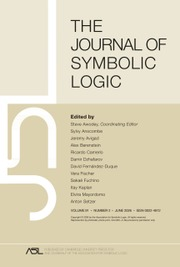The Journal of Symbolic Logic

# SURREAL ORDERED EXPONENTIAL FIELDS

Published online by Cambridge University Press:  13 August 2021

## Abstract

In 2001, the algebraico-tree-theoretic simplicity hierarchical structure of J. H. Conway’s ordered field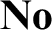${\mathbf {No}}$ of surreal numbers was brought to the fore by the first author and employed to provide necessary and sufficient conditions for an ordered field (ordered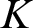$K$ -vector space) to be isomorphic to an initial subfield (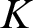$K$ -subspace) of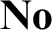${\mathbf {No}}$ , i.e. a subfield (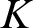$K$ -subspace) of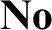${\mathbf {No}}$ that is an initial subtree of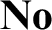${\mathbf {No}}$ . In this sequel, analogous results are established for ordered exponential fields, making use of a slight generalization of Schmeling’s conception of a transseries field. It is further shown that a wide range of ordered exponential fields are isomorphic to initial exponential subfields of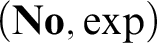$({\mathbf {No}}, \exp )$ . These include all models of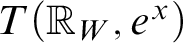$T({\mathbb R}_W, e^x)$ , where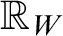${\mathbb R}_W$ is the reals expanded by a convergent Weierstrass system W. Of these, those we call trigonometric-exponential fields are given particular attention. It is shown that the exponential functions on the initial trigonometric-exponential subfields of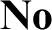${\mathbf {No}}$ , which includes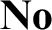${\mathbf {No}}$ itself, extend to canonical exponential functions on their surcomplex counterparts. The image of the canonical map of the ordered exponential field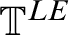${\mathbb T}^{LE}$ of logarithmic-exponential transseries into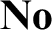${\mathbf {No}}$ is shown to be initial, as are the ordered exponential fields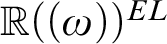${\mathbb R}((\omega ))^{EL}$ and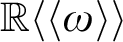${\mathbb R}\langle \langle \omega \rangle \rangle$ .

## MSC classification

Type
Article
Information
The Journal of Symbolic Logic , September 2021 , pp. 1066 - 1115
© Association for Symbolic Logic 2021

## Access options

Get access to the full version of this content by using one of the access options below. (Log in options will check for institutional or personal access. Content may require purchase if you do not have access.)

## Footnotes

*

The in-text reference citations have been corrected. An erratum detailing this update has also been published (doi: 10.1017/jsl.2022.5).

## References

Alling, N., Foundations of Analysis over Surreal Number Fields , North-Holland Publishing Company, Amsterdam, 1987.Google Scholar
Aschenbrenner, M., van den Dries, L. and van der Hoeven, J., Asymptotic Differential Algebra and Model Theory of Transseries, Annals of Mathematics Studies, vol. 195, Princeton University Press, Princeton, 2017.Google Scholar
Aschenbrenner, M., van den Dries, L. and van der Hoeven, J., Numbers, germs and transseries , Proceedings of the International Congress of Mathematicians (B. Sirakov, P. N. de Souza and M. Viana, editors), World Scientific Publishing Company, Hackensack, 2018, pp. 1943.Google Scholar
Aschenbrenner, M., van den Dries, L. and van der Hoeven, J., The surreal numbers as a universal H-field . Journal of the European Mathematical Society , vol. 21 (2019), no. 4, pp. 11791199.CrossRefGoogle Scholar
Bagayoko, V. and van der Hoeven, J., Surreal substructures, Preprint, 2019, https://hal.archives-ouvertes.fr/hal-02151377.Google Scholar
Bagayoko, V. and van der Hoeven, J., The hyperserial field of surreal numbers, Preprint, 2021, https://hal.archives-ouvertes.fr/hal-03232836.Google Scholar
Bagayoko, V., van der Hoeven, J. and Kaplan, E., Hyperserial fields, Preprint, 2021, https://hal.archives-ouvertes.fr/hal-03196388.Google Scholar
Bagayoko, V. and van der Hoeven, J. and Mantova, V., Defining a surreal hyperexponential, Preprint, 2020, https://hal.archives-ouvertes.fr/hal-02861485.Google Scholar
Berarducci, A., Ehrlich, P. and Kuhlmann, S., Mini-workshop: surreal numbers, surreal analysis, Hahn fields and derivations. Abstracts from the mini-workshop held December 18-23, 2016. Oberwolfach Reports, vol. 13 (2016), no. 4, pp. 33133372.10.4171/OWR/2016/60CrossRefGoogle Scholar
Berarducci, A. and Mantova, V., Surreal numbers, derivations and transseries. Journal of the European Mathematical Society (JEMS), vol. 20 (2018), pp. 339390.10.4171/JEMS/769CrossRefGoogle Scholar
Berarducci, A. and Mantova, V., Transseries as germs of surreal functions. Transactions of the American Mathematical Society , vol. 371 (2019), no. 5, pp. 35493592.10.1090/tran/7428CrossRefGoogle Scholar
Conway, J. H., On Numbers and Games , Second ed., A K Peters, Ltd., Natick, Massachusetts, 2001.Google Scholar
Costin, O. and Ehrlich, P., Integration on the surreals: a conjecture of Conway, Kruskal and Norton. Oberwolfach Reports, vol. 13 (2016), no. 4, pp. 33643365.Google Scholar
Costin, O., Ehrlich, P. and Friedman, H., Integration on the surreals: a conjecture of Conway, Kruskal and Norton, Preprint, 2015, arXiv.1334466.Google Scholar
D’Aquino, P., Knight, J., Kuhlmann, S. and Lange, K., Real closed exponential fields . Fundamenta Mathematicae , vol. 219 (2012), pp. 163190.10.4064/fm219-2-6CrossRefGoogle Scholar
Denef, J. and van den Dries, L.,$p$ -adic and real subanalytic sets . Annals of Mathematics (2), vol. 128 (1988), no. 1, pp. 79138.CrossRefGoogle Scholar
Denef, J. and Lipshitz, L., Ultraproducts and approximation in local rings. II . Mathematische Annalen , vol. 253 (1980), pp. 128.10.1007/BF01457817CrossRefGoogle Scholar
van den Dries, L., A generalization of the Tarski-Seidenberg theorem, and some nondefinability results. American Mathematical Society , vol. 15 (1986), pp. 189193.Google Scholar
van den Dries, L., On the elementary theory of restricted elementary functions, this Journal, vol. 53 (1988), pp. 796808.Google Scholar
van den Dries, L. and Ehrlich, P., Fields of surreal numbers and exponentiation . Fundamenta Mathematicae , vol. 167 (2001), pp. 173188; erratum, ibid. 168 (2001), pp. 295–297.CrossRefGoogle Scholar
van den Dries, L., Macintyre, A. and Marker, D., The elementary theory of restricted analytic fields with exponentiation . Annals of Mathematics , vol. 140 (1994), pp. 183205.CrossRefGoogle Scholar
van den Dries, L., Macintyre, A. and Marker, D., Logarithmic-exponential power series . Journal of the London Mathematical Society , vol. 56 (1997), pp. 417434.CrossRefGoogle Scholar
Ehrlich, P., An alternative construction of Conway’s ordered field No . Algebra Universalis , vol. 25 (1988), pp. 716; errata, ibid. 25 (1988), p. 233.10.1007/BF01229956CrossRefGoogle Scholar
Ehrlich, P., Absolutely saturated models . Fundamenta Mathematicae , vol. 133 (1989), pp. 3946.10.4064/fm-133-1-39-46CrossRefGoogle Scholar
Ehrlich, P.. All Number Great and Small, Real Numbers, Generalizations of the Reals, and Theories of Continua (P. Ehrlich, editor), Netherlands, Kluwer Academic Publishers, Dordrecht, 1994, pp. 239258.CrossRefGoogle Scholar
Ehrlich, P., Number systems with simplicity hierarchies: A generalization of Conway’s theory of surreal numbers, this Journal, vol. 66 (2001), pp. 12311258. Corrigendum, this Journal, vol. 70 (2005), p. 1022.Google Scholar
Ehrlich, P., Surreal numbers: An alternative construction (abstract). The Bulletin of Symbolic Logic, vol. 8 (2002), p. 448.Google Scholar
Ehrlich, P., Conway names, the simplicity hierarchy and the surreal number tree . Journal of Logic and Analysis , vol. 3 (2011), no. 1, pp. 126.Google Scholar
Ehrlich, P., The absolute arithmetic continuum and the unification of all numbers great and small . The Bulletin of Symbolic Logic , vol. 18 (2012), pp. 145.10.2178/bsl/1327328438CrossRefGoogle Scholar
Ehrlich, P., Surreal ordered exponential fields . Oberwolfach Reports , vol. 13 (2016), no. 4, pp. 33243325.Google Scholar
Ehrlich, P. and Kaplan, E., Number systems with simplicity hierarchies: A generalization of Conway’s theory of surreal numbers II, this Journal, vol. 83 (2018), pp. 617633.Google Scholar
Fornasiero, A., Integration on surreal numbers, Ph.D. thesis, University of Edinburgh, 2004.Google Scholar
Fornasiero, A., Embedding Henselian fields into power series . Journal of Algebra , vol. 304 (2006), pp. 112156.CrossRefGoogle Scholar
Fornasiero, A., Initial embeddings in$\mathbf{No}$ of models of${T}_{\mathrm{an}}\left({exp}\right)$ , 2013.Google Scholar
Gabrielov, A., Projections of semianalytic sets. Funkcional. Anal. i Priložen., vol. 2 (1968), no. 4, pp. 1830.Google Scholar
Gonshor, H., An Introduction to the Theory of Surreal Numbers, Cambridge University Press, Cambridge, 1986.10.1017/CBO9780511629143CrossRefGoogle Scholar
Hahn, H., Über die nichtarchimedischen Grössensysteme, Sitzungsberichte der Kaiserlichen Akademie der Wissenschaften, Wien, Mathematisch-Naturwissenschaftliche Klasse 116 (Abteilung IIa) (1907), pp. 601655.Google Scholar
van der Hoeven, J., Asymptotique Automatique, PhD thesis, École Polytechnique, 1997.Google Scholar
Kuhlmann, F.-V., Kuhlmann, S. and Shelah, S., Exponentiation in power series fields. Proceedings of the American Mathematical Society, vol. 125 (1997), no. 11, pp. 31773183.CrossRefGoogle Scholar
Kuhlmann, S., Ordered Exponential Fields , Fields Institute Monographs, 12, American Mathematical Society, Providence, 2000.Google Scholar
Kuhlmann, S. and Shelah, S.,$\kappa$ -bounded exponential-logarithmic power series fields , Annals of Pure and Applied Logic , vol. 136 (2005), pp. 284296.Google Scholar
Kuhlmann, S. and Tressl, M., Comparison of exponential-logarithmic and logarithmic-exponential series. Mathematical Logic Quarterly , vol. 58 (2012), pp. 434448.CrossRefGoogle Scholar
Mendelson, E., An Introduction to Mathematical Logic , fifth ed., CRC Press, Boca Raton, 2010.Google Scholar
Mourgues, M.-H. and Ressayre, J.-P., Every real closed field has an integer part, this Journal, vol. 58 (1993), pp. 641647.Google Scholar
Neumann, B. H., On ordered division rings . Transactions of the American Mathematical Society, vol. 66 (1949), pp. 202252.CrossRefGoogle Scholar
Ressayre, J.-P., Integer parts of real closed exponential fields , Arithmetic, Proof Theory, and Computational Complexity (Clote, P. and Krajicek, J., editors), Clarendon Press, Oxford, 1993, pp. 278288.Google Scholar
Schmeling, M., Corps de transséries, PhD thesis, Université de Paris 7, 2001.Google Scholar
Sfouli, H., On the elementary theory of restricted real and imaginary parts of holomorphic functions . Notre Dame Journal of Formal Logic , vol. 53 (2012), no. 1, pp. 6777.CrossRefGoogle Scholar
Siegel, A., Combinatorial Game Theory , American Mathematical Society, Providence, 2013.CrossRefGoogle Scholar

1
Cited by

# Save article to Kindle

Note you can select to save to either the @free.kindle.com or @kindle.com variations. ‘@free.kindle.com’ emails are free but can only be saved to your device when it is connected to wi-fi. ‘@kindle.com’ emails can be delivered even when you are not connected to wi-fi, but note that service fees apply.

Find out more about the Kindle Personal Document Service.

SURREAL ORDERED EXPONENTIAL FIELDS
Available formats
×

# Save article to Dropbox

To save this article to your Dropbox account, please select one or more formats and confirm that you agree to abide by our usage policies. If this is the first time you used this feature, you will be asked to authorise Cambridge Core to connect with your Dropbox account. Find out more about saving content to Dropbox.

SURREAL ORDERED EXPONENTIAL FIELDS
Available formats
×

# Save article to Google Drive

To save this article to your Google Drive account, please select one or more formats and confirm that you agree to abide by our usage policies. If this is the first time you used this feature, you will be asked to authorise Cambridge Core to connect with your Google Drive account. Find out more about saving content to Google Drive.

SURREAL ORDERED EXPONENTIAL FIELDS
Available formats
×
×